### Area Of Plane Figures Class 8th Mathematics AP Board Solution

##### Question 1.A rectangular acrylic sheet is 36 cm by 25 cm. From it, 56 circular buttons, each of diameter 3.5 cm have been cut out. Find the area of the remaining sheet.Answer: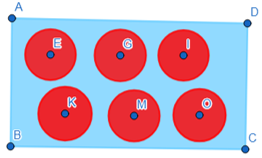The dimensions of rectangular sheet are 36 cm by 25 cm.The Area of rectangular sheet = 36 × 25 = 900 sq.cmFrom this sheet, 56 circular buttons are cut as shown,Diameter of each circle = 3.5 cm⇒ radius of each button, r = 1.75 cmArea of one button = πr2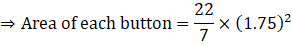⇒ Area of each button = 9.625 sq.cmTotal area of buttons cut = area of each button × 56⇒ Total Area of buttons = 539 sq. cmArea of remaining sheet (area shaded with blue) = (Area of rectangular sheet) – (total area of buttons)⇒ Req. area = 900 – 539 = 361 sq.cm∴ Area of the remaining sheet is 361 sq.cmQuestion 2.Find the area of a circle inscribed in a square of side 28 cm. [Hint: Diameter of the circle is equal to the side of the square]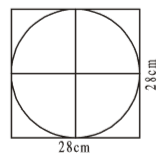Answer:Since the circle is inscribed in a square of side 28 cm, this means that the diameter of the circle is equal to the side of square.Diameter of circle, d = 28cm⇒ Radius of circle, r = 14 cmAs we know that,Area of a circle = π × r2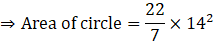⇒ Area of circle = 616 sq.cm∴ The area of circle inscribed in the square is 616 sq.cmQuestion 3.Find the area of the shaded region in each of the following figures.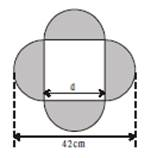[Hint: d +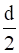+=42]d = 21∴ side of the square 21 cmAnswer:The shaded region is formed of four semicircles and as the base of these semicircles lie on the side of a square, therefore the diameter of the semicircle is equal to the side of the square.⇒ diameter of semicircle = side of square = d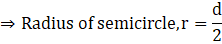It is shown that, r + d + r = 42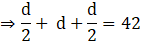⇒ d = 21 cm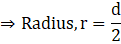⇒ Radius, r = 10.5 cm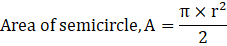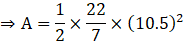⇒ A = 173.25 sq.cmArea of shaded region = 4 × (Area of semicircle)⇒ 4 × A⇒ 4 × 173.25⇒ 693 sq.cm∴ Area of the shaded region is 693 sq.cmQuestion 4.Find the area of the shaded region in each of the following figures.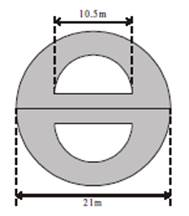Answer:Diameter of bigger semicircle, D = 21 cm⇒ Radius of bigger semicircle, R = D÷ 2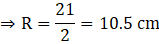Diameter of smaller semicircle, d = 21 cm⇒ Radius of smaller semicircle, r = d÷ 2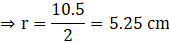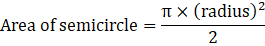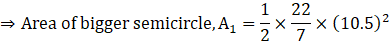⇒ A1 = 173.25 sq.cmSimilarly,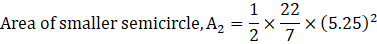⇒ A2 = 43.31 sq.cmArea of shaded region = 2 × (A1 – A2)⇒ Req. area = 2 × (173.25 – 43.31)⇒ 2 × 129.94⇒ 259.87 sq.cm∴ Area of shaded region is 259.87 sq.cmQuestion 5.The adjacent figure consists of four small semi-circles of equal radii and two big semi-circles of equal radii (each 42 cm). Find the area of the shaded region.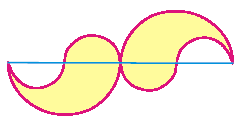Answer:As we know that,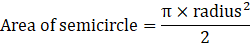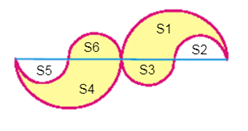From the above figure we see,Diameter of bigger semi-circle, D = 42 cm⇒ S1 and S4 are bigger semicircles with Radius, R = 21 cmAlso, S2, S3, S5 and S6 are smaller semi-circles with diameter, d equal to the radius of bigger semi-circle.⇒ d = R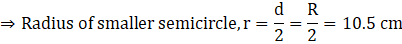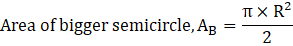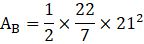⇒ AB = 693 sq.cmSimilarly,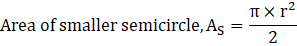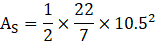⇒ AS = 173.25 sq.cmArea of shaded region = Area(S1) + Area(S4) + Area(S3) + Area(S6) – Area (S2) – Area(S5)⇒ Area of shaded region = AB + AB + AS + AS – AS - AS⇒ Area = 2 × AB⇒ 2 × 693 = 1386 sq.cm∴ Area of shaded region is 1386 sq.cmQuestion 6.The adjacent figure consists of four half circles and two quarter circles. If OA = OB = OC = OD = 14 cm. Find the area of the shaded region.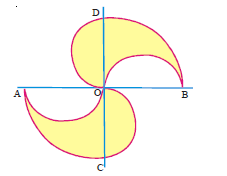Answer:Let the area of a semicircle be AS and the area of a quadrant be AQSince there are four semi-circles, out of which two are shaded and two are unshaded, therefore the area of shaded region is equal to:Since the area of shaded region is equal on both sides of line AOB,So, if we calculate area of upper portion, then we can simply twice that area in order to get required area.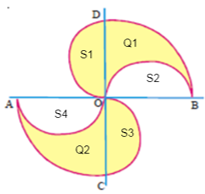In figure above, S1, S2, S3,and S4 represents semi-circles and Q1 and Q2 represents quadrants.⇒ Area of shaded region = 2 × (area of upper shaded portion on line AOB)⇒ Area of shaded region = 2 × [area(S1) + area(Q1) – area(S2)]As S1 and S2 are equal because of same radius, therefore their area will also be equal i.e area(S1) = area(S2)⇒ Area of shaded region = 2 × area(Q1)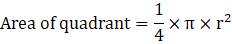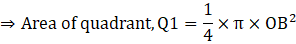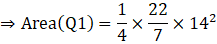⇒ Area (Q1) = 154 sq.cm⇒ Required area = 2 × area(Q1)⇒ 2 × 154 = 308 sq.cm∴ Area of shaded region is 308 sq.cmQuestion 7.In adjacent figure A, B, C and D are centers of equal circles which touch externally in pairs and ABCD is a square of side 7 cm. Find the area of the shaded region.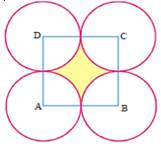Answer:Side of square, a = 7cmArea of square, AS = a2⇒ AS = 72 = 49 sq.cm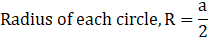⇒ R = 3.5 cmThere is a quadrant of each of four circles that is present inside the square,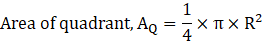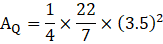⇒ AQ = 9.625 sq.cmShaded area = Area(square) – [4 × area(quadrant)]⇒ Shaded area = AS – [4 × AQ]⇒ 49 – (4 × 9.625)⇒ 49 – 38.5⇒ 10.5 sq.cm∴ Area of shaded region is 10.5 sq.cmQuestion 8.The area of an equilateral triangle is 49√3 cm2. Taking each angular point as centre, a circle is described with radius equal to half the length of the side of the triangle as shown in the figure. Find the area of the portion in the triangle not included in the circles.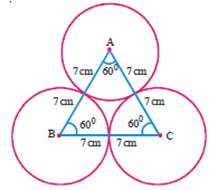Answer:Let the side of the triangle be ‘a’As we know that,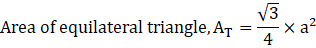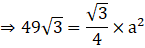⇒ a = 14 cmRadius of each circle, r = a ÷ 2⇒ r = 7 cm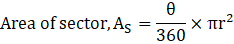Here, θ = 60°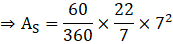⇒ AS = 25.67 sq.cmRequired area = Area(Δ ABC) – 3× areaof sector⇒ Req. area = 49√3 – [3× 25.67]⇒ 84.87 – 77.01⇒ 7.86 sq.cm∴ Area of the portion in the triangle not included in the circles is 7.86 sq.cmQuestion 9.Four equal circles, each of radius ‘a’ touch one another. Find the area between them.Answer:Radius of each circle, r = a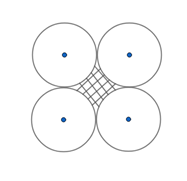We need to find the shaded area,If we connect the four centers we will get a square of side 2a.Area of square,AS = (2a)2 = 4a2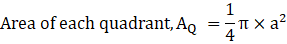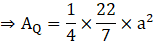Area of shaded region = AS – [4 × AQ]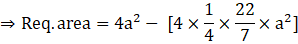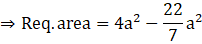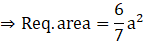Question 10.Four equal circles are described about the four corners of a square so that each circle touches two of the others. Find the area of the space enclosed between the circumferences of the circles, each side of the square measuring 14 cm.Answer:Since each side of square measures 14 cm, so the radius of each circle is half of the side.Therefore, radius of circle, R = 7 cm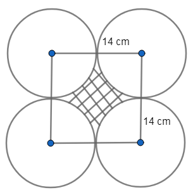We need to find the area of the shaded region,There is a quadrant of each of four circles that is present inside the square,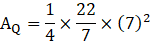⇒ AQ = 38.5 sq.cmAlso, area of square, AS = 142 = 196 sq.cmArea of shaded region = AS – [4 × AQ]⇒ 196 – [4 × 38.5]⇒ 196 – 154⇒ 42 sq.cm∴ area of shaded region is 42 sq. cmQuestion 11.From a piece of cardboard, in the shape of a trapezium ABCD, and AB||CD and ∠BCD = 900, quarter circle is removed. Given AB = BC = 3.5cm and DE = 2cm. Calculate the area of the remaining piece of the cardboard. (Take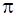to be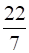)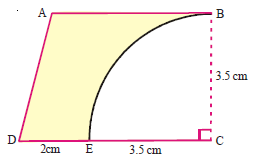Answer:AB = BC = 3.5 cm and DE = 2cm⇒ DC = DE + EC = 2 + 3.5 = 5.5 cmAs we know that,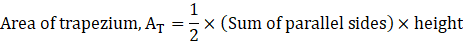Height of trapezium here, BC = 3.5 cm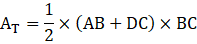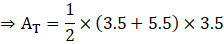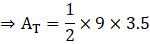⇒ AT = 15.75 sq.cmNow, area of quarter circle = AQ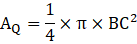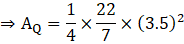⇒ AQ = 9.625 sq.cmRequired area = AT – AQ⇒ Req. area = 15.75 – 9.625 = 6.125 sq.cm∴ Area of remaining piece of cardboard is 6.125 sq.cmQuestion 12.A horse is placed for grazing inside a rectangular field 70m by 52 m and is tethered to one corner by a rope 21 m long. How much area can it graze?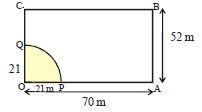Answer:The length of rope to which horse is tied will be equal to the radius of the quarter circle that the horse grazes.⇒ Radius, r = 21mAs we know that,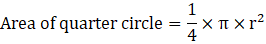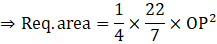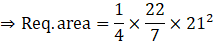⇒ Area = 346.5 sq.m∴ The area that horse can graze is 346.5 m2

PDF FILE TO YOUR EMAIL IMMEDIATELY PURCHASE NOTES & PAPER SOLUTION. @ Rs. 50/- each (GST extra)

HINDI ENTIRE PAPER SOLUTION

MARATHI PAPER SOLUTION

SSC MATHS I PAPER SOLUTION

SSC MATHS II PAPER SOLUTION

SSC SCIENCE I PAPER SOLUTION

SSC SCIENCE II PAPER SOLUTION

SSC ENGLISH PAPER SOLUTION

SSC & HSC ENGLISH WRITING SKILL

HSC ACCOUNTS NOTES

HSC OCM NOTES

HSC ECONOMICS NOTES

HSC SECRETARIAL PRACTICE NOTES

# 2019 Board Paper Solution

HSC ENGLISH SET A 2019 21st February, 2019

HSC ENGLISH SET B 2019 21st February, 2019

HSC ENGLISH SET C 2019 21st February, 2019

HSC ENGLISH SET D 2019 21st February, 2019

SECRETARIAL PRACTICE (S.P) 2019 25th February, 2019

HSC XII PHYSICS 2019 25th February, 2019

CHEMISTRY XII HSC SOLUTION 27th, February, 2019

OCM PAPER SOLUTION 2019 27th, February, 2019

HSC MATHS PAPER SOLUTION COMMERCE, 2nd March, 2019

HSC MATHS PAPER SOLUTION SCIENCE 2nd, March, 2019

SSC ENGLISH STD 10 5TH MARCH, 2019.

HSC XII ACCOUNTS 2019 6th March, 2019

HSC XII BIOLOGY 2019 6TH March, 2019

HSC XII ECONOMICS 9Th March 2019

SSC Maths I March 2019 Solution 10th Standard11th, March, 2019

SSC MATHS II MARCH 2019 SOLUTION 10TH STD.13th March, 2019

SSC SCIENCE I MARCH 2019 SOLUTION 10TH STD. 15th March, 2019.

SSC SCIENCE II MARCH 2019 SOLUTION 10TH STD. 18th March, 2019.

SSC SOCIAL SCIENCE I MARCH 2019 SOLUTION20th March, 2019

SSC SOCIAL SCIENCE II MARCH 2019 SOLUTION, 22nd March, 2019

XII CBSE - BOARD - MARCH - 2019 ENGLISH - QP + SOLUTIONS, 2nd March, 2019

# HSCMaharashtraBoardPapers2020

(Std 12th English Medium)

HSC ECONOMICS MARCH 2020

HSC OCM MARCH 2020

HSC ACCOUNTS MARCH 2020

HSC S.P. MARCH 2020

HSC ENGLISH MARCH 2020

HSC HINDI MARCH 2020

HSC MARATHI MARCH 2020

HSC MATHS MARCH 2020

# SSCMaharashtraBoardPapers2020

(Std 10th English Medium)

English MARCH 2020

HindI MARCH 2020

Hindi (Composite) MARCH 2020

Marathi MARCH 2020

Mathematics (Paper 1) MARCH 2020

Mathematics (Paper 2) MARCH 2020

Sanskrit MARCH 2020

Sanskrit (Composite) MARCH 2020

Science (Paper 1) MARCH 2020

Science (Paper 2)

Geography Model Set 1 2020-2021

MUST REMEMBER THINGS on the day of Exam

Are you prepared? for English Grammar in Board Exam.

Paper Presentation In Board Exam

How to Score Good Marks in SSC Board Exams

Tips To Score More Than 90% Marks In 12th Board Exam

How to write English exams?

How to prepare for board exam when less time is left

How to memorise what you learn for board exam

No. 1 Simple Hack, you can try out, in preparing for Board Exam

How to Study for CBSE Class 10 Board Exams Subject Wise Tips?

JEE Main 2020 Registration Process – Exam Pattern & Important Dates

NEET UG 2020 Registration Process Exam Pattern & Important Dates

How can One Prepare for two Competitive Exams at the same time?

8 Proven Tips to Handle Anxiety before Exams!